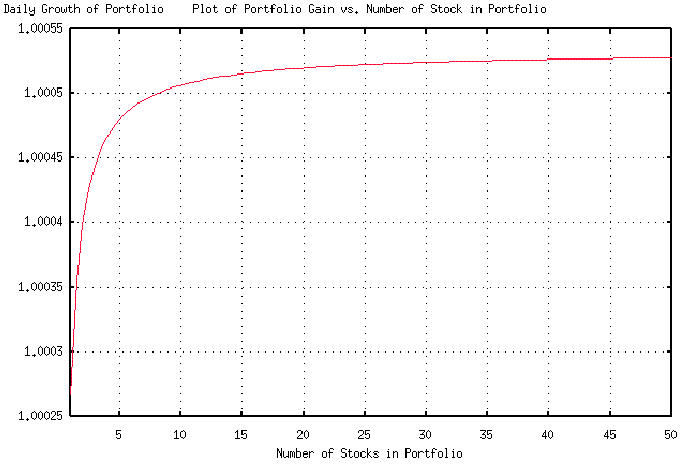# Re: forwarded message from William F. Hummel

From: John Conover <john@email.johncon.com>
Subject: Re: forwarded message from William F. Hummel
Date: Fri, 14 Aug 1998 22:24:49 -0700

```John Conover writes:
>
> If you look at the equation for P and G, as a function of n, you will
> find that it has a shape like a square root function-there is little
> advantage in holding more than 10 stocks, (although there is a grave
> eleventh stock is modestly marginal.
>

Attached is a plot of the daily growth in value of a portfolio as a
function of the number of stocks in the portfolio. It uses the stock
from the stock exchange model developed previously, where:

rms = 0.0231

avg = 0.0005331

P = 0.511555

and:

G = ((1 + (0.0231 / sqrt (x)))
** ((((0.0005331 / 0.0231) * sqrt (x)) + 1) / 2)) *
((1 - (0.0231 / sqrt (x))) **
((1 - ((0.0005331 / 0.0231) * sqrt (x))) / 2))

stocks in the portfolio, but significant disadvantage in less.

John

``````
--

John Conover, john@email.johncon.com, http://www.johncon.com/

```